## WhatIs.com.com

### logic gate (AND, OR, XOR, NOT, NAND, NOR and XNOR)

#### By TechTarget Contributor

A logic gate is a device that acts as a building block for digital circuits. They perform basic logical functions that are fundamental to digital circuits. Most electronic devices we use today will have some form of logic gates in them. For example, logic gates can be used in technologies such as smartphones, tablets or within memory devices.

In a circuit, logic gates will make decisions based on a combination of digital signals coming from its inputs. Most logic gates have two inputs and one output. Logic gates are based on Boolean algebra. At any given moment, every terminal is in one of the two binary conditions, false or true. False represents 0, and true represents 1. Depending on the type of logic gate being used and the combination of inputs, the binary output will differ. A logic gate can be thought of like a light switch, wherein one position the output is off -- 0, and in another, it is on -- 1. Logic gates are commonly used in integrated circuits (IC).

### Basic logic gates

There are seven basic logic gates: AND, OR, XOR, NOT, NAND, NOR, and XNOR.

AND | OR | XOR | NOT | NAND | NOR | XNOR

The AND gate is so named because, if 0 is called "false" and 1 is called "true," the gate acts in the same way as the logical "and" operator. The following illustration and table show the circuit symbol and logic combinations for an AND gate. (In the symbol, the input terminals are at left and the output terminal is at right.) The output is "true" when both inputs are "true." Otherwise, the output is "false." In other words, the output is 1 only when both inputs one AND two are 1.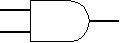AND gate

 Input 1 Input 2 Output 1 1 1 1 1

The OR gate gets its name from the fact that it behaves after the fashion of the logical inclusive "or." The output is "true" if either or both of the inputs are "true." If both inputs are "false," then the output is "false." In other words, for the output to be 1, at least input one OR two must be 1.OR gate

 Input 1 Input 2 Output 1 1 1 1 1 1 1

The XOR ( exclusive-OR ) gate acts in the same way as the logical "either/or." The output is "true" if either, but not both, of the inputs are "true." The output is "false" if both inputs are "false" or if both inputs are "true." Another way of looking at this circuit is to observe that the output is 1 if the inputs are different, but 0 if the inputs are the same.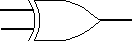XOR gate

 Input 1 Input 2 Output 1 1 1 1 1 1

A logical inverter, sometimes called a NOT gate to differentiate it from other types of electronic inverter devices, has only one input. It reverses the logic state. If the input is 1, then the output is 0. If the input is 0, then the output is 1.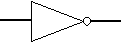Inverter or NOT gate
 Input Output 1 1

The NAND gate operates as an AND gate followed by a NOT gate. It acts in the manner of the logical operation "and" followed by negation. The output is "false" if both inputs are "true." Otherwise, the output is "true."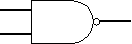NAND gate

 Input 1 Input 2 Output 1 1 1 1 1 1 1

The NOR gate is a combination OR gate followed by an inverter. Its output is "true" if both inputs are "false." Otherwise, the output is "false."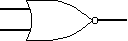NOR gate

 Input 1 Input 2 Output 1 1 1 1 1

The XNOR (exclusive-NOR) gate is a combination XOR gate followed by an inverter. Its output is "true" if the inputs are the same, and "false" if the inputs are different.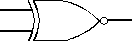XNOR gate

 Input 1 Input 2 Output 1 1 1 1 1 1

Complex operations can be performed using combinations of these logic gates. In theory, there is no limit to the number of gates that can be arrayed together in a single device. But in practice, there is a limit to the number of gates that can be packed into a given physical space. Arrays of logic gates are found in digital ICs. As IC technology advances, the required physical volume for each individual logic gate decreases and digital devices of the same or smaller size become capable of performing ever-more-complicated operations at ever-increasing speeds.

### Composition of logic gates

High or low binary conditions are represented by different voltage levels. The logic state of a terminal can, and generally does, often change as the circuit processes data. In most logic gates, the low state is approximately zero volts (0 V), while the high state is approximately five volts positive (+5 V).

Logic gates can be made of resistors and transistors or diodes. A resistor can commonly be used as a pull-up or pull-down resistor. Pull-up and pull-down resistors are used when there are any unused logic gate inputs to connect to a logic level 1 or 0. This prevents any false switching of the gate. Pull-up resistors are connected to Vcc (+5V), and pull-down resistors are connected to ground (0 V).

Commonly used logic gates are TTL and CMOS. TTL, or Transistor-Transistor Logic, ICs will use NPN and PNP type Bipolar Junction Transistors. CMOS, or Complementary Metal-Oxide-Silicon, ICs are constructed from MOSFET or JFET type Field Effect Transistors. TTL IC's may commonly be labeled as the 7400 series of chips, while CMOS ICs may often be marked as a 4000 series of chips.

16 Dec 2020